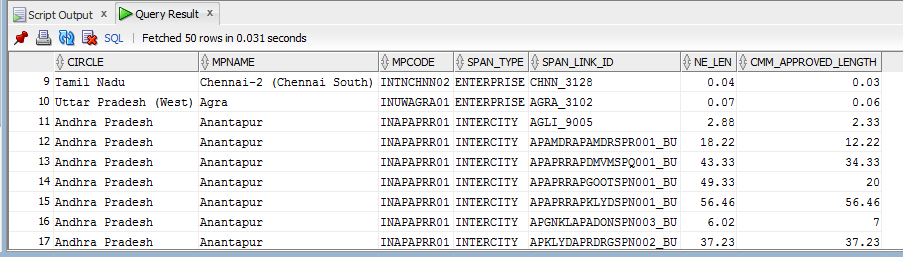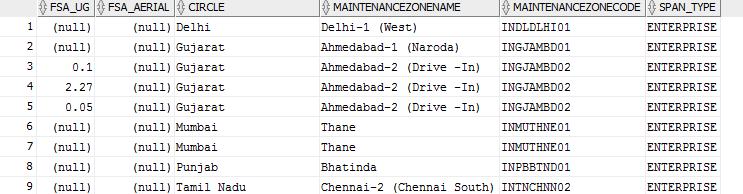# #StackBounty: #oracle #stored-procedures Update multiple columns based on percentage wise calculation in Oracle

### Bounty: 50

NOTE: Updating total question with sample data and output.

I need to update some columns by comparing from another table and update the columns percentage wise. So here I go.

first of all , the query to get CMM approved length is below with other columns which I need

``````select CIRCLE,regexp_substr(MP,'[^/]+',1,1)MPNAME,regexp_substr(MP,'[^/]+',1,2)MPCODE,
ROUTE_APPROVED_BY_CMM as CMM_APPROVED_LENGTH from
TBL_FIBER_INV_CMP_REPORT_MV
where CMM_APPROVED_DATE IS NOT NULL OR ROUTE_APPROVED_BY_CMM > 0 OR
JOB_PROGRESS_FLAG = 1;
``````

and the output of above query looks like this below imageNow comes the second part which is calculation of the percentage wise ratio

CASE 1: If The CMM approved length in above image is 70km and NE length from (NE,UG, AR len from `NE.MV_SPAN@DB_LINK_NE_VIEWER`)comes out to be 100Km then divide `(UG=80Km and AR=20Km)`

Then percentage of bifurcation would be 80% UG_length and 20% AR_length. So, against 70km of CMM approved kilometer, `TBL_FIBER_INV_SIGN_OFF_SHEET` should update the column as `UG = 56Km` and `AR = 14Km`.
the bifurcation should be updated in below table `TBL_FIBER_INV_SIGN_OFF_SHEET`

and the table sample data looks like below:-CASE 2: IF `CMM approved length` and `NE length` is same. for ex: 70km (UG = 60 Km and AR=10Km) then in `TBL_FIBER_INV_SIGN_OFF_SHEET` table should update UG = `60Km and AR = 10Km`.

Below are the table description of both the tables.

``````Table:- NE.MV_SPAN@DB_LINK_NE_VIEWER

Name                           Null Type
------------------------------ ---- --------------
OBJECTID                            NUMBER
SPAN_NAME                           VARCHAR2(20)
CATEGORY_NAME                       VARCHAR2(20)
TYPE_NAME                           VARCHAR2(20)
SPAN_REF_NAME                       VARCHAR2(20)
RJ_NETWORK_ENTITY_ID                VARCHAR2(39)
RJ_SPAN_RJID                        VARCHAR2(50)
RJ_ROUTE_NAME                       VARCHAR2(200)
RJ_NETWORK_CATEGORY                 VARCHAR2(50)
RJ_SPAN_ID                          VARCHAR2(25)
RJ_STATE_NAME                       VARCHAR2(50)
RJ_STATE_CODE                       VARCHAR2(2)
RJ_R4G_STATE_NAME                   VARCHAR2(50)
RJ_R4G_STATE_CODE                   VARCHAR2(2)
RJ_MAINTENANCE_ZONE_NAME            VARCHAR2(50)
RJ_MAINTENANCE_ZONE_CODE            VARCHAR2(25)
RJ_CONSTRUCTION_METHODOLOGY         VARCHAR2(50)
SHAPE                               ST_GEOMETRY()
CALCULATED_LENGTH                   NUMBER(38,8)

Table:- TBL_FIBER_INV_SIGN_OFF_SHEET

Name                Null Type
------------------- ---- --------------
JOB_ID                   NUMBER
CIRCLE                   NVARCHAR2(100)
MAINTENANCEZONENAME      NVARCHAR2(100)
MAINTENANCEZONECODE      NVARCHAR2(50)
SPAN_TYPE                NVARCHAR2(20)
NE_LENGTH                NUMBER
HOTOOFFERDATE            DATE
FSA_UG                   NUMBER
FSA_AERIAL               NUMBER    ``````

UPDATE To get UG_length and AR_length from `NE.MV_SPAN@DB_LINK_NE_VIEWER` here is the logic

``````SELECT ROUND(SUM(NVL(CALCULATED_LENGTH,0)/1000),4) AS NE_LENGTH,
ROUND(SUM(CASE WHEN RJ_CONSTRUCTION_METHODOLOGY NOT LIKE '%AERIAL%' OR RJ_CONSTRUCTION_METHODOLOGY IS  NULL THEN NVL(CALCULATED_LENGTH,0) ELSE 0 END)/1000,4) AS UG_LENGTH
,ROUND(SUM(CASE WHEN RJ_CONSTRUCTION_METHODOLOGY LIKE '%AERIAL%' THEN NVL(CALCULATED_LENGTH,0) ELSE 0 END)/1000,4) AS AR_LENGTH
``````

Also some common columns from all the tables are

`SPAN_LINK_ID` from `TBL_FIBER_INV_SIGN_OFF_SHEET` table

`SPAN_LINK_ID` from `TBL_FIBER_INV_CMP_REPORT_MV` table

AND

`RJ_LINK_ID`, `RJ_SPAN_ID`, `CALCULATED_LENGTH`, `RJ_CONSTRUCTION_METHODOLOGY` FROM `NE.MV_SPAN@DB_LINK_NE_VIEWER` table

THE LOGIC WHICH I TRIED TILL NOW

``````CREATE OR REPLACE PROCEDURE UPD_UG_AR_BY_CMM AS
BEGIN

for cur_r in (
select circle,
regexp_substr(MP,'[^/]+',1,1)MAINTENANCE_ZONE_NAME,
regexp_substr(MP,'[^/]+',1,2)MAINTENANCE_ZONE_CODE,
SPAN_TYPE,
NE_LENGTH,
ROUTE_APPROVED_BY_CMM
from TBL_FIBER_INV_CMP_REPORT_MV
where CMM_APPROVED_DATE IS NOT NULL
OR ROUTE_APPROVED_BY_CMM > 0
OR JOB_PROGRESS_FLAG = 1
)

LOOP

IF cur_r.ROUTE_APPROVED_BY_CMM >  SELECT ROUND(SUM(NVL(CALCULATED_LENGTH,0)/1000),4) AS NE_LENGTH,
ROUND(SUM(CASE WHEN RJ_CONSTRUCTION_METHODOLOGY NOT LIKE '%AERIAL%' OR RJ_CONSTRUCTION_METHODOLOGY IS  NULL THEN NVL(CALCULATED_LENGTH,0) ELSE 0 END)/1000,4) AS UG_LENGTH
,ROUND(SUM(CASE WHEN RJ_CONSTRUCTION_METHODOLOGY LIKE '%AERIAL%' THEN NVL(CALCULATED_LENGTH,0) ELSE 0 END)/1000,4) AS AR_LENGTH
THEN

BEGIN
UPDATE TBL_FIBER_INV_SIGN_OFF_SHEET
SET  FSA_UG = UG_LENGTH,  --- divide 80% of NE_LENGTH
FSA_AERIAL = AR_LENGTH    --- divide 20% of NE_LENGTH

END

NULL;
END UPD_UG_AR_BY_CMM;``````

but iam stuck at calculation of percentage wise ratio.

Get this bounty!!!

This site uses Akismet to reduce spam. Learn how your comment data is processed.